Custom Formulas

This powerful app lets you create your own custom formulas and then perform computations using them by prompting you for the input values. This app is easy to use, but unlike simpler apps, multiple entered values can be fed into multiple formulas and multiple output values can be displayed. The output of one formula in a group can be fed into the next by using the same variable name.

Related formulas can be grouped into categories to make them easy to find. e.g. Maths formulas, Surveying formulas, Loan interest formulas etc.

The order of variables displayed to the user can be changed, as can the number of decimal digits of precision shown in the output fields. Three example formulas are pre-installed with the app. In order of increasing complexity they are: slope percentage, compound interest, and point scale factor.

What’s New
4.0: Changed the math constant “e” to be “e” to avoid confusion when you want to use the letter “e” as a variable. Added the ability to export all formulas, or all formulas within a category in one go.

Screenshots

••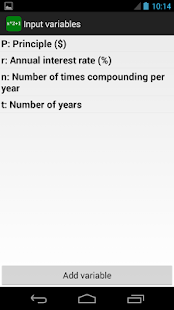•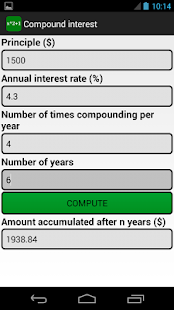•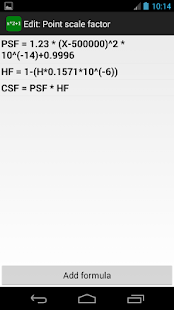•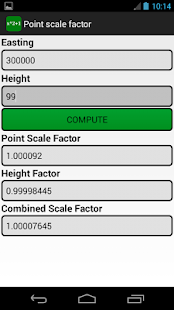•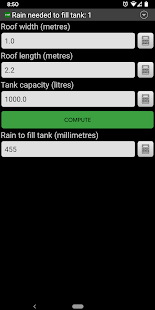••